Wir verwenden Google für unsere Suche. Mit Klick auf „Suche aktivieren“ aktivieren Sie das Suchfeld und akzeptieren die Nutzungsbedingungen.

# New in SG-Lib 3.4

###### PLoutercontour(CPL,out)- returns a point list that has no crossings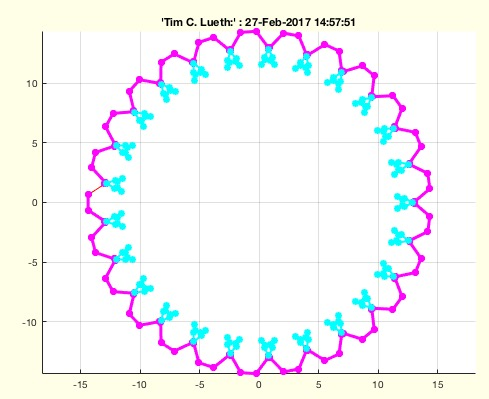% PLoutercontour(CPL,out) - returns a point list that has no crossings% (by Tim Lueth and Jelena Prsa, VLFL-Lib, 2017-FEB-27 as class: CLOSED % POLYGON LISTS)% % In some cases, a fnctn such as CPLgrow creates cross that cross itself. % In this case, cw-oriented CPLs should return only the outer parts of % the contour and ccw-oriented CPLs should return the inner part of the % contour; The direction is detected automatically but can be overwritten % by the parameter out. Out=true => the outer contour is returned. % Out=false => the inner contour is returned. (Status of: 2017-02-28)% % See also: CPLoutercontour, CPLgrow% % [PLN,PLX]=PLoutercontour([CPL,out])% === INPUT PARAMETERS ===% CPL: Point List% out: returns the FIRST outer contour if true; if false the first inner; % default is true% === OUTPUT RESULTS ======% PLN: Single Point List of the FIRST outer contour% PLX: Singe Point List of the FIRST outer contour% % EXAMPLE: % PLoutercontour(CPLgrow(CPLofPL(PLgearDIN(0.5,25)),-0.3),false);%
###### TregisterConvexHull(VLA,VLB)- returns the registration matrix based on the convex hull of the vertices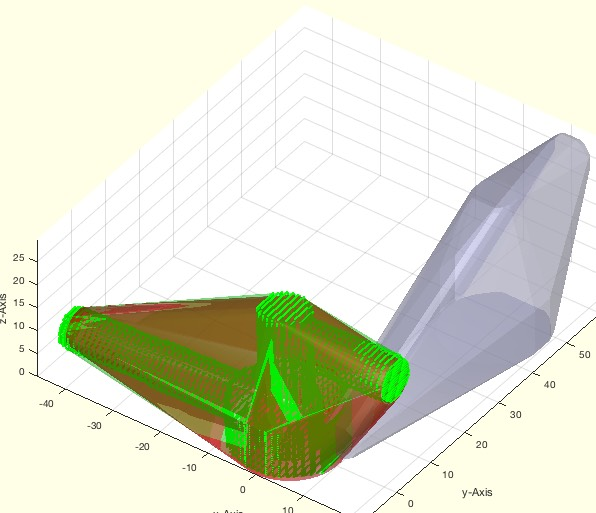% TregisterConvexHull(VLA,VLB) - returns the registration matrix based on the convex hull of the vertices% (by Tim Lueth, VLFL-Lib, 2017-FEB-24 as class: ANALYTICAL GEOMETRY)% % This fnctn is just a simple fnctn for registering voxel models with % their original Solid Geoemtry (Status of: 2017-02-24)% % T=TregisterConvexHull(VLA,VLB)% === INPUT PARAMETERS ===% VLA: Vertex list A or Solid A% VLB: Vertex list B or Solid B% === OUTPUT RESULTS ======% T: Transformation from B into A% % EXAMPLE: Show the result of a random geoemtry% T=TofR(rot(pi/3,pi,6,pi/9,[10 10 10])), inv(T), VL=rand(10,3); % TregisterConvexHull(VL,VLtransT(VL,T))% %
###### VMofSG(SG,n,adjxy,shsl)- returns a voxel model of a Solid Geoemtry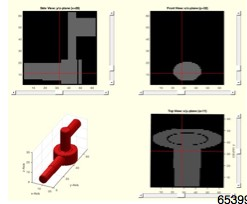% VMofSG(SG,n,adjxy,shsl) - returns a voxel model of a Solid Geoemtry% (by Tim Lueth, VLFL-Lib, 2017-FEB-23 as class: VOXELS)% % The voxel size is adjusted automatically to maximize the resolution. % The absolute position in space is lost. The orientation is unchanged. % (Status of: 2017-02-27)% % See also: SGofVMdelaunay, SGofVMmarchcube, VMplot% % [VM,vs,SGN]=VMofSG([SG,n,adjxy,shsl])% === INPUT PARAMETERS ===% SG: Solid Geometry% n: Voxel size vector; default is [64 64 64]% adjxy: adjust x and y size; default is false % shsl: delay per slice if progress should be shown% === OUTPUT RESULTS ======% VM: Voxel Model% vs: Voxel size% SGN: Optional Solid by marching cube%
###### VMplot (IM,p,SG)- plots a 3D volumetric matrix in 2x2 view% VMplot (IM,p,SG) - plots a 3D volumetric matrix in 2x2 view% (by Tim Lueth, NAV-Lib, 2017-FEB-23 as class: VISUALIZATION)% % Based on VMplot_2012. Does support sliders for scrolling, and 3D % visualization;% % Global VMplotPos specifies the cross hair position% (Status of: 2017-04-02)% % See also: VMintensityscale, VMimage, VMimrot90, VMmontage, VMpseudo3D, % VMreaddicom, VMreaddicomdir, VMuidicom, VMgetSubplot, VMginput% % VMplot([IM,p,SG])% === INPUT PARAMETERS ===% IM: 3D Matrix % p: optional point of interest; default is center% SG: optional solid of the Volume;% % EXAMPLE: % [V,vs]=VMreaddicomdir('/Volumes/LUETH-WIN/WIN AIM Matlab % Libraries/VLFL-Lib/AIM_DICOMFILES');% [V,vs]=VMresize(V,vs,vs);% SG=SGofVMdelaunay(V>1400,vs);% VMplot(V,'',SG)% %
###### pixelofaxis- returns the axis position of the current axis in the figure% pixelofaxis - returns the axis position of the current axis in the figure% (by Tim Lueth, VLFL-Lib, 2017-FEB-23 as class: USER INTERFACE)% % This fnctn is useful for placing uicontrol objects relative to subplots % such as in VMplot (Status of: 2017-02-24)% % See also: uipanel, uicontrol, pixelpositionofP, vertexpicker, select3d, % getpixelposition% % sp=pixelofaxis% === OUTPUT RESULTS ======% sp: [left bottom size-x size-y]% % EXAMPLE: % subplot(2,2,4);% sp=pixelofaxis% uicontrol('Style', 'popup','String', % {'R-Joint','P-Joint','G-Joint'},'Position', sp)%
###### SGsurfaceplot(SG,SIL,si)- plots the separated surfaces of SG in different colors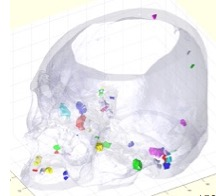% SGsurfaceplot(SG,SIL,si) - plots the separated surfaces of SG in different colors% (by Tim Lueth, VLFL-Lib, 2017-FEB-22 as class: SURFACES)% % See also: SGfigure, SGplot, SGseparate, SGsurfaces, SGsurfacehistogram, % SGwriteSeparatedSTL, SGpuzzlecut3D% % [SG,SIL]=SGsurfaceplot(SG,[SIL,si])% === INPUT PARAMETERS ===% SG: Solid Geometry% SIL: Surface index list decending sorted by number of facets;% si: solid index for plot % === OUTPUT RESULTS ======% SG: Solid Geometry% SIL: Surface Index List; created if missing%
###### camset (p,d,ez)- sets the camera target to a specific point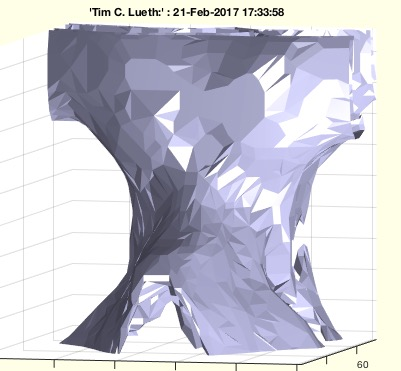% camset (p,d,ez) - sets the camera target to a specific point% (by Tim Lueth, VLFL-Lib, 2017-FEB-21 as class: USER INTERFACE)% % if no position is specified, the user will be requested to select a % point by a cross hair (Status of: 2017-02-21)% % See also: zoommpatch, camplot, tcamera% % camset([p,d,ez])% === INPUT PARAMETERS ===% p: point; default is ''; % d: diameter of axis% ez: direction to camera position;% % EXAMPLE: % camset ('',100); camplot;%
###### camplot- plots a line of the current camera position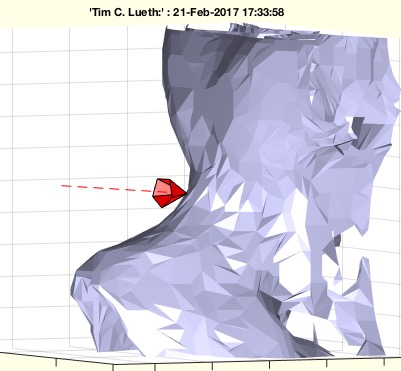% camplot - plots a line of the current camera position% (by Tim Lueth, VLFL-Lib, 2017-FEB-21 as class: USER INTERFACE)% % See also: zoommpatch, camset, tcamera% % [p0,p1,v,a]=camplot% === OUTPUT RESULTS ======% p0: CameraPosition% p1: CameraTarget% v: CameraViewAngle% a: axis%
###### ROBgetJointPosition(KGID,jset)- returns the position of a set of joints of a robot% ROBgetJointPosition(KGID,jset) - returns the position of a set of joints of a robot% (by Tim Lueth, RT-Lib, 2017-FEB-21 as class: KINEMATICS AND FRAMES)% % Procedure example for Samuel Detzel% The Kinematic Group ID is an identifier for a set of structural % connected joints such as a serial link robot arm% t0 = the time of the controller's internal clock when receiving the % ROBgetJointPosition command% t1 = the time of the controller's internal clock when sending the % ROBgetJointPosition results (Status of: 2017-02-22)% % [t,qi]=ROBgetJointPosition(KGID,[jset])% === INPUT PARAMETERS ===% KGID: Kinematic Group ID% jset: Joint set; defualt is empty == all joints of KGID% === OUTPUT RESULTS ======% t: time vector (t0 t1)% qi: joint values of all or the requested set if joints [q1 q2 ]%
###### exp_2017_02_21- EXPERIMENT SCRIPT to load and precalcuate a skull bone surface model% exp_2017_02_21 - EXPERIMENT SCRIPT to load and precalcuate a skull bone surface model% (by Tim Lueth, VLFL-Lib, 2017-FEB-21 as class: EXPERIMENTS)% % exp_2017_02_21%
###### selectCameraPosition (p)- selects and sets the camera position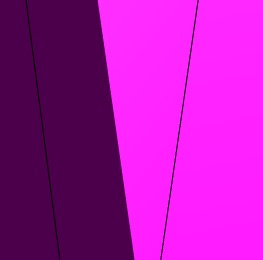% selectCameraPosition (p) - selects and sets the camera position% (by Tim Lueth, VLFL-Lib, 2017-FEB-21 as class: USER INTERFACE)% % selectCameraPosition([p])% === INPUT PARAMETERS ===% p: point to set; default is empty%
###### SGofVMdelaunay(V,vs,s)- returns a surface model for a delaunay reconstructed volume model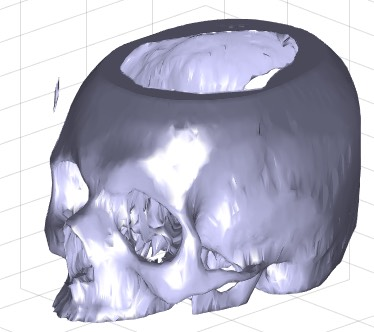% SGofVMdelaunay(V,vs,s) - returns a surface model for a delaunay reconstructed volume model% (by Tim Lueth, VLFL-Lib, 2017-FEB-17 as class: VOXELS)% % This fnctn takes about 20 second for a 256 x 256 x 100 voxel model. % (Status of: 2017-02-18)% % See also: VLFLofVMdelaunay, VLFLofVMmarchcube, TR3ofVM, SGofVMmarchcube% % [SG,TR4]=SGofVMdelaunay(V,[vs,s])% === INPUT PARAMETERS ===% V: Voxel Model (~=0 is reconstruction)% vs: voxel size, optional% s: step size% === OUTPUT RESULTS ======% SG: Solid Geoemtry% TR4: Delaunay tetrahedron model% % EXAMPLE: % [V,vs]=VMreaddicomdir('AIM_DICOMFILES');% [a,as]=VMresize(V,[0.5 0.5 0.5],vs);% SG=SGofVMdelaunay(a>1400,as);% SGcut(SG,40);% c=CPLofSGslice3(SG,20);% PLFLofCPLdelaunay(c);% PLFLofCPLpoly(c)%
###### SGofVMmarchcube(V,vs,cl)- returns a surface model by the marching cubes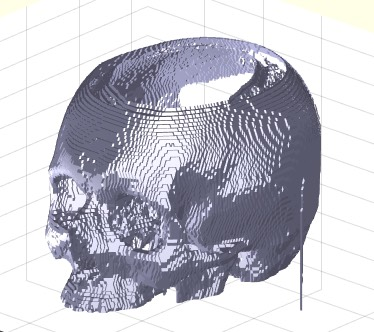% SGofVMmarchcube(V,vs,cl) - returns a surface model by the marching cubes% (by Tim Lueth, VLFL-Lib, 2017-FEB-17 as class: VOXELS)% % This fnctn uses the MarchingCubes fnctn from Matlab Central written by % Peter Hammer in 2011 based on the Octave fnctn written by Martin Helm % (www.mhelm.de/octave/m/marching_cube.m) in 2009. It contains also code % from Oliver Woodford for removing duplicated vertices. (Status of: % 2017-02-23)% % See also: VLFLofVMdelaunay, VLFLofVMmarchcube, TR3ofVM, SGofVMdelaunay% % SG=SGofVMmarchcube(V,[vs,cl])% === INPUT PARAMETERS ===% V: Logical Volume Model% vs: voxel size, optional% cl: closes open facets by adding empty planes; default is true% === OUTPUT RESULTS ======% SG: Vertex list% % EXAMPLE: [V,vs]=VMreaddicomdir('AIM_DICOMFILES');% [a,as]=VMresize(V,[0.5 0.5 0.5],vs);% SG=SGofVMmarchcube(a>1400,as);% SGcut(SG,40);% c=CPLofSGslice3(SG,20);% PLFLofCPLdelaunay(c);% PLFLofCPLpoly(c)%
###### VMresize(VM,dm,vs)- resizes a voxel image model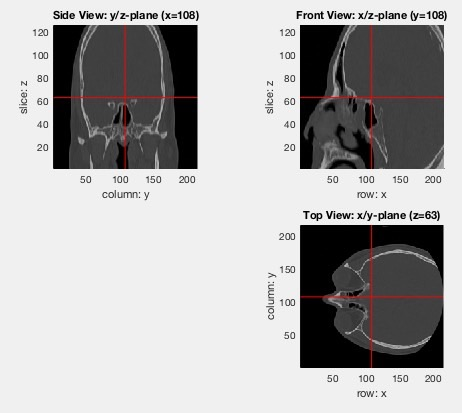% VMresize(VM,dm,vs) - resizes a voxel image model% (by Tim Lueth, VLFL-Lib, 2017-FEB-17 as class: VOXELS)% % [V,vs]=[V,vs,vs]); returns voxels of same size 1 mm x 1mm x 1mm% [V,vs]=[V,vs); returns voxels of same size 1 mm x 1mm x 1mm% (Status of: 2017-02-17)% % See also: VMreaddicomdir, VMmontage, VMplot, VMintensityscale% % [FVM,vs]=VMresize(VM,[dm,vs])% === INPUT PARAMETERS ===% VM: voxel mode; [X x Y x Z]% dm: dimension either scale [0.5 0.5 0.5] or vs% vs: optional voxel size for rescaling; default is dm% === OUTPUT RESULTS ======% FVM: New Voxel Model with new dimensions% vs: Voxel size after resizing% % EXAMPLE: % [V,vs]=VMreaddicomdir('AIM_DICOMFILES');% VMresize(V,vs,vs); % voxel size = 1 mm% VMresize(V,vs/2,vs); % voxel size = 2 mm%
###### SGgethandle(SG)- returns a handle if the object is already drawn in gca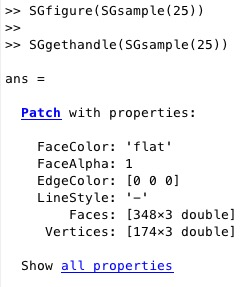% SGgethandle(SG) - returns a handle if the object is already drawn in gca% (by Tim Lueth, VLFL-Lib, 2017-FEB-14 as class: USER INTERFACE)% % Does not support all kinds of SG yet.% Is more a VLFLgethandle fnctn (Status of: 2017-02-14)% % See also: SGplot% % h=SGgethandle(SG)% === INPUT PARAMETERS ===% SG: Solid Geometry% === OUTPUT RESULTS ======% h: handle%
###### selectV3d(SG)- plots a SG in a figure and shows selected normal vectors of a selected corner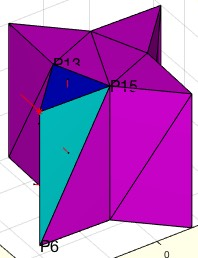% selectV3d(SG) - plots a SG in a figure and shows selected normal vectors of a selected corner% (by Tim Lueth, VLFL-Lib, 2017-FEB-14 as class: USER INTERFACE)% % See also: select3d, vertexpicker% % selectV3d([SG])% === INPUT PARAMETERS ===% SG: Solid Geometry to plot%
###### FLselect(FL,vi)- returns all neighbor facets of vi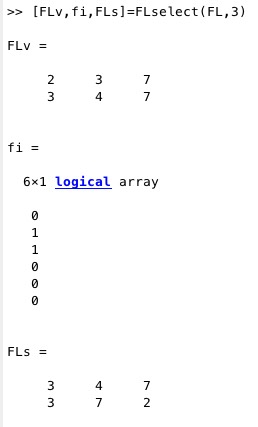% FLselect(FL,vi) - returns all neighbor facets of vi% (by Tim Lueth, VLFL-Lib, 2017-FEB-14 as class: AUXILIARY PROCEDURES)% % Some fnctns such as MLofSG process a complete solid. Nevertheless, % finally, only facets that are linked to a vertex are required. FLselect % returns form a FL the index of all facts that contain vi, the reduced % facet list, and a facet list that is sorted in a way that the FL starts % always with vi% (Status of: 2017-02-14)% % [FLv,fi,FLs]=FLselect(FL,vi)% === INPUT PARAMETERS ===% FL: Facet list% vi: index of vertex% === OUTPUT RESULTS ======% FLv: reduced facet list% fi: facet index of FL% FLs: FL with sortet rows% % EXAMPLE: % [VL,FL]=VLFLcone(30,'',6)% [FLv,fi,FLs]=FLselect(FL,3)%
###### VLbreakEdges(VL,R)- returns a vertex list (VL) with broken edges% VLbreakEdges(VL,R) - returns a vertex list (VL) with broken edges % (by Tim Lueth, VLFL-Lib, 2017-FEB-13 as class: ANALYZING PROCEDURES)% % Replaces edges by a simple shortcut. The desired radius can be reduced % automatically if necessary. It is possible to maximize the radius % automatically.% In case that R is negative; VLradialEdges is used instead (Status of: % 2017-02-13)% % See also: PLradialEdges, SGcontourtube, RLofEulerInterpolation, % VLinsertEulerSteps, VLradialEdges, TofPez, PLtangentcirc, % VLtangentcirc, SGbreakCorners, SGradialCorners% % NVL=VLbreakEdges(VL,[R])% === INPUT PARAMETERS ===% VL: Vertex list nx3% R: Radius; default is 1% === OUTPUT RESULTS ======% NVL: New vertex list% % EXAMPLE: % VL=roundn(rand(6,3)*100,5)% VLbreakEdges(VL,2)% VLbreakEdges([0 0 0;0 0 100; 100 0 100;100 100 100]); view(0,0)% VLbreakEdges([0 0 0;0 0 100; 100 0 0],10); view(0,0)%
###### SGcone(H,R,nf)- returns a solid geometry of a cone% SGcone(H,R,nf) - returns a solid geometry of a cone% (by Tim Lueth, VLFL-Lib, 2017-FEB-13 as class: MODELING PROCEDURES)% % uses VLFLcone; (Status of: 2017-02-13)% % See also: VLFLcone, SGsphere, SGbox, SGlinkage, SGtext% % [SG,FL,FLb]=SGcone(H,[R,nf])% === INPUT PARAMETERS ===% H: Height% R: Radius; default is H/2% nf: Number of facets, default is 16% === OUTPUT RESULTS ======% SG: Vertex list% FL: Facet list of the cone% FLb: Facet list of the bottom%
###### exp_2017_02_13 (A,vi,R)- Experiment for analyzing normal vectors of facets and corners and edges% exp_2017_02_13 (A,vi,R) - Experiment for analyzing normal vectors of facets and corners and edges% (by Tim Lueth, VLFL-Lib, 2017-FEB-13 as class: EXPERIMENTS)% % Around mid Feb, im getting tired to reimplement CAD fnctns that I just % need to convince students/researcher that are used to use CATIA instead % of improving design processes (Status of: 2017-02-14)% % exp_2017_02_13([A,vi,R])% === INPUT PARAMETERS ===% A: Solid Geoemtry% vi: vertex of interest (corner); 0 == select graphically% R: Optional Radius%
###### FLofDelaunay(VL,vni)- returns the convex hull of a vertex list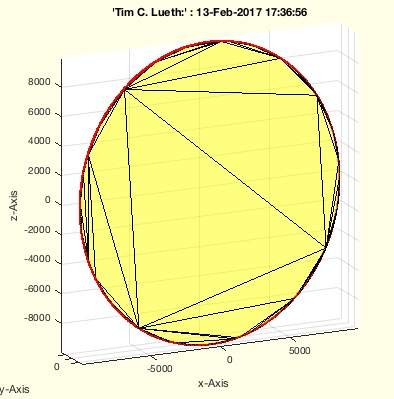% FLofDelaunay(VL,vni) - returns the convex hull of a vertex list% (by Tim Lueth, VLFL-Lib, 2017-FEB-12 as class: SURFACES)% % works with 2D and 3D and plane surfaces in 3D% By intention, matlab's delaunayTriangulation does not return a % tesselation for 3D coordinates if all vertices are in one plane since % in 3D the fnctns returns a tetrahedron tesselation. In this case, a % transformation matrix is calculated from the vertex list, the vertex % list is transformed into a point list, and a 2D tesselation is % returned. SOMETIMES, delaunayTriangulation, returns also a tesselation % for planar structures. THis has to be detected in future (Status of: % 2017-02-12)% % FL=FLofDelaunay(VL,[vni])% === INPUT PARAMETERS ===% VL: Vertex list (nx2) or (nx3)% vni: optional index of VL% === OUTPUT RESULTS ======% FL: Facet list to close VL(vni,:)% % EXAMPLE: % FLofDelaunay(VLtransR(VLaddz(PLstar(10,30),30),rot(pi/3,pi/3,pi/3)))% FLofDelaunay(rand(100,3)*100-50)%
###### SGradialCorners(A,vi,R)- should breaks corners and edges of solids% SGradialCorners(A,vi,R) - should breaks corners and edges of solids% (by Tim Lueth, VLFL-Lib, 2017-FEB-12 as class: SURFACES)% % If vi==0, the user should select interactively a vertex% First all patches/plane surfaces connected to an corner are calculated. % For the further calculation only the vertices are used that define the % outer limits of the face. This fnctn uses VLradialEdges to replace the % original edges at a corned by a corved convex element. Completely new % version on 2017-20-12 based on exp_2017_02_12.% (Status of: 2017-02-13)% % See also: SGbreakCorners, VLradialEdges% % A=SGradialCorners([A,vi,R])% === INPUT PARAMETERS ===% A: Solid Geometry% vi: list of corned index (n) or corner vertex list (nx3); default is ''% R: radius; default is 1mm% === OUTPUT RESULTS ======% A: Solid Geometry with rounded corners%
###### findVL(NVL,VL,ai,thr)- returns the indices of a list in another list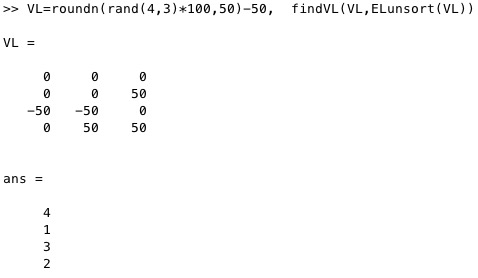% findVL(NVL,VL,ai,thr) - returns the indices of a list in another list% (by Tim Lueth, VLFL-Lib, 2017-FEB-12 as class: AUXILIARY PROCEDURES)% % This fnctn finds the indices of some vertices in a longer list. For a % complete remapping use fnctn "maprows". % bi=findVL(VL,ai); bi=ai(bi) (Status of: 2017-02-13)% % See also: maprows, reversesortindex% % bi=findVL(NVL,VL,[ai,thr])% === INPUT PARAMETERS ===% NVL: Long vertex list to search in% VL: Second vertex list to find in NVL% ai: optional index list to search for % thr: threshold for find; default is 1e-12% === OUTPUT RESULTS ======% bi: index in NVL % % EXAMPLE: % VL=roundn(rand(20,3)*100,5)-50% findVL(VL,unique(VL,'rows'))%
###### exp_2017_02_12(A,vi,R)- Experiment to show the breaking of vertices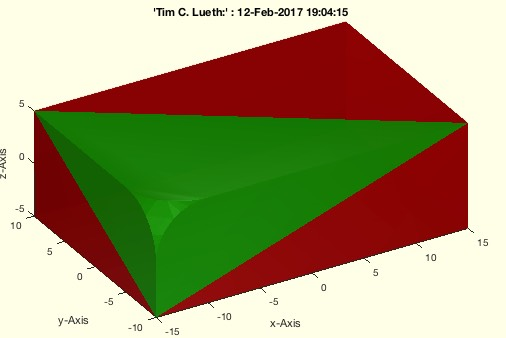% exp_2017_02_12(A,vi,R) - Experiment to show the breaking of vertices% (by Tim Lueth, VLFL-Lib, 2017-FEB-12 as class: EXPERIMENTS)% % First all patches/plane surfaces connected to an corner are calculated. % For the further calculation only the vertices are used that define the % outer limits of the surface.% This fnctn uses VLradialEdges to replace the original edges at a corned % by a corved convex element. (Status of: 2017-02-12)% % See also: SGbreakvertices, SGradialCorners, exp_2017_02_01% % A=exp_2017_02_12([A,vi,R])% === INPUT PARAMETERS ===% A: Solid Geoemtry% vi: list of corned index (n) or corner vertex list (nx3); default is 4% R: radius; default is 1mm% === OUTPUT RESULTS ======% A: Solid Geoemtry with rounded corners%
###### Tof2vec(p1,p2,q1,q2)- returns transformation matrix based on the 2 point movements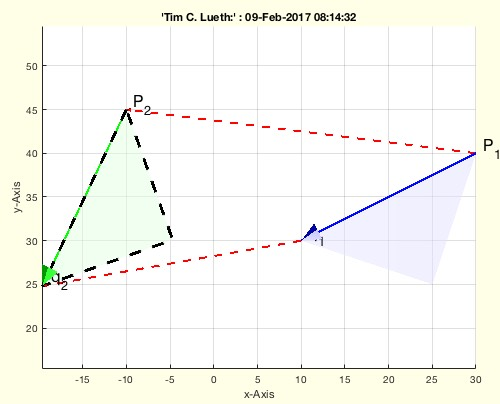% Tof2vec(p1,p2,q1,q2) - returns transformation matrix based on the 2 point movements% (by Tim Lueth, VLFL-Lib, 2017-FEB-08 as class: ANALYTICAL GEOMETRY)% % This fnctn helps to transform points from one coordinate system into % another based on two known point movements. P1 is the center of the 1st % and P2 is the center of the second system.% W_T_P1 and W_T_P2 ==> P2_T_P1 ==> P2_T_P1 * P1_P (Status of: % 2017-02-09)% % See also: TofR, TofVL, TPL, TofDPhiH, T3ofT2, T3P, T2P, TofP, TofPez, % TofPEul% % [T12,T21]=Tof2vec(p1,p2,q1,q2)% === INPUT PARAMETERS ===% p1: 1st point before movement% p2: 1st point after movement% q1: 2nd point before movement% q2: 2nd point before movement% === OUTPUT RESULTS ======% T12: 2_T_1 Transformation from T1 into T2% T21: 1_T_2 Transformation from T2 into T1% % EXAMPLE: % Tof2vec([0 10],[-10 10],[0 15],[-20 10])%
###### CPLfaceplot(PL,c,w,a)- plots the faces of a CPL% CPLfaceplot(PL,c,w,a) - plots the faces of a CPL% (by Tim Lueth, VLFL-Lib, 2017-FEB-08 as class: CLOSED POLYGON LISTS)% % See also: VLELplots, CPLplot, PLplot, VLplot% % h=CPLfaceplot(PL,[c,w,a])% === INPUT PARAMETERS ===% PL: Point List or CPL% c: color% w: countour width% a: alpha; default is 0.5% === OUTPUT RESULTS ======% h: handle% % EXAMPLE: % SGfigure; % CPLfaceplot([PLcircle(20);NaN NaN; [0 0; 10 0; 5 5]],'b',0.1)%
###### textP(p,str,delx,dely,Fnts)- draws a texlabel text at the position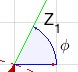% textP(p,str,delx,dely,Fnts) - draws a texlabel text at the position% (by Tim Lueth, VLFL-Lib, 2017-FEB-07 as class: ANALYTICAL GEOMETRY)% % See also: textT, textVL, textCVL% % h=textP(p,str,[delx,dely,Fnts])% === INPUT PARAMETERS ===% p: point% str: texlabel% delx: displacement x; default is 0.02 (2%)% dely: displacement y; default is 0.02 (2%)% Fnts: Fontsize; default is 16% === OUTPUT RESULTS ======% h: handle to text%
###### exp_2017_02_07-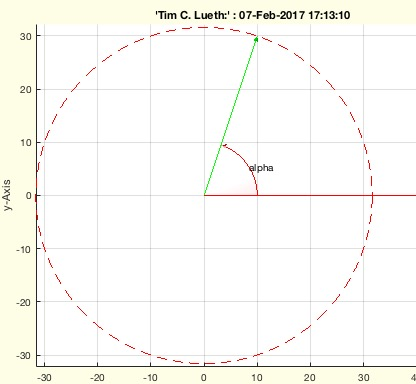% exp_2017_02_07 - % (by Tim Lueth, VLFL-Lib, 2017-FEB-07 as class: ANALYTICAL GEOMETRY)% % exp_2017_02_07%
###### aplot(pc,la,lb,c,w,r,alpha)- draws an angle relative to a center and two vectors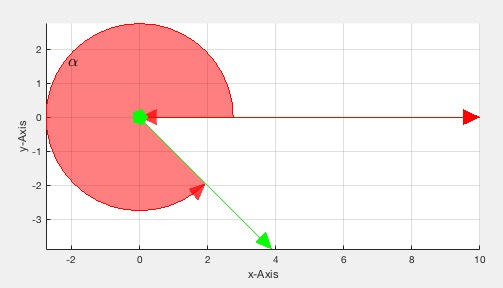% aplot(pc,la,lb,c,w,r,alpha) - draws an angle relative to a center and two vectors% (by Tim Lueth, VLFL-Lib, 2017-FEB-07 as class: ANALYTICAL GEOMETRY)% % See also: aplot, pplot, lplot, tfplot, tlplot, slplot, plotTP, plotL, % plotT, T2Plot, textP, textT, textVL% % [h,pt,a]=aplot(pc,la,[lb,c,w,r,alpha])% === INPUT PARAMETERS ===% pc: center of angle% la: 1st vector% lb: 2nd vector or angle or rotation matrix% c: color; default is 'r'% w: width of lines; default is 1;% r: radius; default is % alpha: transparency% === OUTPUT RESULTS ======% h: handle to all elements of the angle drawing% pt: point for text desciption% a: angle that was used if lb was a vector% % EXAMPLE: % [h,pt]=aplot ([0 0],[10 0],3.5*pi/2)% textP(pt,'alpha');%
###### pixelpositionofP(p)- returns the pixelposition of a point coordinate% pixelpositionofP(p) - returns the pixelposition of a point coordinate% (by Tim Lueth, VLFL-Lib, 2017-FEB-06 as class: USER INTERFACE)% % If uipanels or uicontrols should be placed at point coordinates, the % position of a point coordinate relative to the figure is requested.% It does not work correctly yet for axis equal;% Panels are moved correctly, but not fixed pixel positions (Status of: % 2017-03-19)% % See also: uipanel, uicontrol, vertexpicker, select3d, getpixelposition, % pixelofaxis% % [pf,ppf,ppa]=pixelpositionofP(p)% === INPUT PARAMETERS ===% p: point coordinate [x y]% === OUTPUT RESULTS ======% pf: pixel coordinate in figure (uicontrol)% ppf: pixel coordinate in percentage of figure (uipanel)% ppa: pixel coordinate in percentage of axis% % EXAMPLE: set a uipanel at 0.8 0.1% figure; axis on; grid on; axis equal% pixelpositionofP([0.8 0.1])% %% SGmodelLink4(L,L1,L2,K,H) - Creates Solid for a Link im Style "Link4"% (by Tim Lueth, VLFL-Lib, 2017-FEB-05 as class: MODELING PROCEDURES)% % Notation style% 0= same level% +n = n* Height H above z plane% -n = n* height H under z plane% Knob Position% 'BU' = Base frame upper side% 'BL' = Base frame lower sider% 'BU' = Follower Frame upper side% 'BL' = Follower Frame lower sider (Status of: 2017-02-05)% % See also: SGmodelLink, SGmodelJoint, SGmodelNode, SGmodelLink3, % SGmodelKeyhole, SGmodelLink1, SGmodelLink2, SGmodelLink3% % [SG,SGA,SGB]=SGmodelLink4([L,L1,L2,K,H])% === INPUT PARAMETERS ===% L: Length% L1: Level of Frame B; default is 0% L2: Level of Frame F; default is 1% K: Knob position; default is '' % H: Height of link; default is 9 % === OUTPUT RESULTS ======% SG: Solid Geoemtry including Frames% SGA: Left joint of the link% SGB: Right joint of the link% % EXAMPLE: % A=SGmodelLink4('',0,2);SGaddrelSG(A,A,'alignT',{'B','F'});% A=SGmodelLink4('',0,1);SGaddrelSG(A,A,'alignT',{'B','F'});% A=SGmodelLink4('',0,-1,'BL,FU');SGaddrelSG(A,A,'alignT',{'B','F'});% %
###### SGmodelSliderhole(RA,RO,lw,LA,LO,HO,slot,WI)- Creates a SG including a key and a keyhole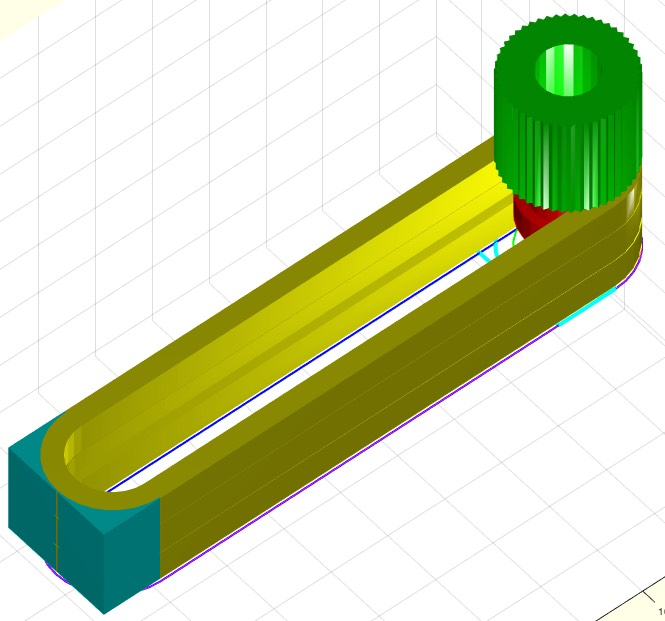% SGmodelSliderhole(RA,RO,lw,LA,LO,HO,slot,WI) - Creates a SG including a key and a keyhole% (by Tim Lueth, VLFL-Lib, 2017-FEB-05 as class: MODELING PROCEDURES)% % SG modeling fnctn to create revolute joint that can be printed % assembled or separated and can be lined on an axis (Status of: % 2017-02-05)% % See also: SGmodelLink, SGmodelJoint, SGmodelNode, SGmodelLink3, % SGmodelKeyhole, SGmodelLink1, SGmodelLink2% % [SGK,SGH,SGP,SGT,SGB]=SGmodelSliderhole([RA,RO,lw,LA,LO,HO,slot,WI])% === INPUT PARAMETERS ===% RA: Axle Radius% RO: Outer Radius% lw: Length of sliding distance% LA: [L1 L2 L3] describing key length% LO: [L1 L2 L3] describing key hole length% HO: Width of a flange in distance RO; default is 2*RO% slot: default is 0.3 mm (SLS & SLA)% WI: Inner Wall Thickness% === OUTPUT RESULTS ======% SGK: Solid Geoemtry of Key% SGH: Solid Geoemtry of Keyhole% SGP: Solid Geometry of flange plate% SGT: Optional Knob on the upper side; separated from SGK% SGB: Optional Knob on the lower side; separated from SGK% % EXAMPLE: Print job was successful on Form2 SLA-Printer on 2017-02-05% SGmodelSliderhole(3,6,'',9)% [A,B,C,D]=SGmodelSliderhole(3,6,'',9);% SGanalyzeGroupParts({A,B,C,D})% %
###### PLcircleoval(R,n,L,Ri)- returns a circle-based-oval point-list% PLcircleoval(R,n,L,Ri) - returns a circle-based-oval point-list% (by Tim Lueth, VLFL-Lib, 2017-FEB-05 as class: ANALYTICAL GEOMETRY)% % Introduced first in SolidGeometry 3.4% % See also: PLcircle, PLcircseg, PLevolvente, PLgearDIN, PLhelix, % PLkidney, PLrand, PLspiral, PLsquare, PLstar% % PL=PLcircleoval([R,n,L,Ri])% === INPUT PARAMETERS ===% R: Radius% n: optional number of points for half circle% L: Length; default is 0;% Ri: optional inner Radius for links; default is 0% === OUTPUT RESULTS ======% PL: Point List% % EXAMPLE: % SGfigure; CPLplot(PLcircleoval(10,'',60,5),'r-',2)% % See also: PLcircle, PLcircseg, PLevolvente, PLgearDIN, PLhelix, % PLkidney, PLrand, PLspiral, PLsquare, PLstar%
###### PLofCPL(CPL)- converts a nested CPL into a nested PL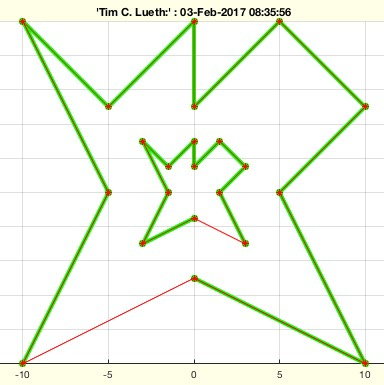% PLofCPL(CPL) - converts a nested CPL into a nested PL% (by Tim Lueth, VLFL-Lib, 2017-FEB-01 as class: CLOSED POLYGON LISTS)% % This fnctn is useful if CPLS are extruded to solids. CPLs are contours % that are separted by NaN NaN. Nevertheless; sometimes the first point % is doubled at the end, somethimes not. This fnctn returns CPLs that are % separated by NaN NaN but the point lists are open% Works with PL and VL (Status of: 2017-02-03)% % See also: CPLofPL% % NPL=PLofCPL(CPL)% === INPUT PARAMETERS ===% CPL: Closed polygon list with unclear closing condition% === OUTPUT RESULTS ======% NPL: Closed polygon list with open polygons% % EXAMPLE: Try% PLofCPL([PLcircle(5);NaN NaN;PLcircle(3)]) % There are no changes% PLofCPL(CPLsample(7)) % remove the closing lines% PLofCPL(VLaddz(CPLsample(7))) % Now try with VL%
###### centerPL(PL)- returns the mean of the convex hull of the point list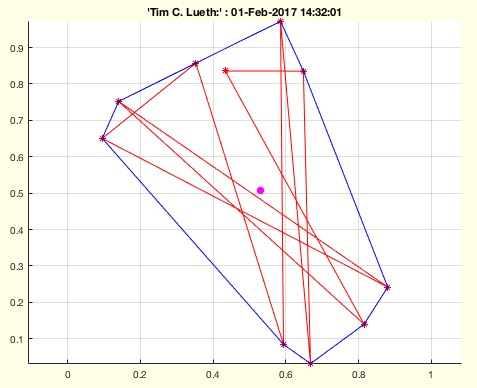% centerPL(PL) - returns the mean of the convex hull of the point list% (by Tim Lueth, VLFL-Lib, 2017-FEB-01 as class: ANALYTICAL GEOMETRY)% % See also: center3P, center4P, centerVL% % pc=centerPL(PL)% === INPUT PARAMETERS ===% PL: Point list (n x 2)% === OUTPUT RESULTS ======% pc: point list center% % EXAMPLE: try:% centerPL(rand(10,2))%
###### exp_2017_02_01- Experiment to show the breaking of vertices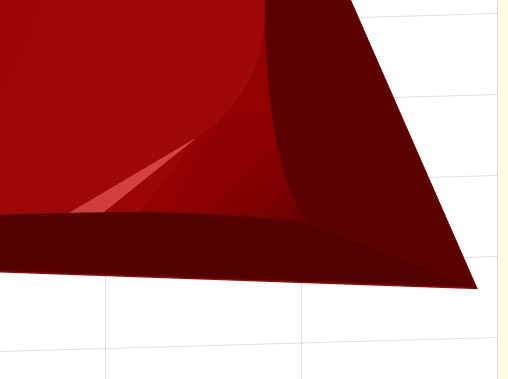% exp_2017_02_01 - Experiment to show the breaking of vertices% (by Tim Lueth, VLFL-Lib, 2017-FEB-01 as class: EXPERIMENTS)% % This fnctn uses VLradialEdges to replace the original edges at a corned % by a corved convex element.% There is still a problem if more than one point is linked to the corner % for the same plane surface (Status of: 2017-02-03)% % See also: SGbreakvertices, SGradialCorners% % exp_2017_02_01%
###### woNaN(PL)- returns a row list without all rows that have a NaN in first row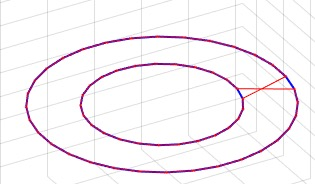% woNaN(PL) - returns a row list without all rows that have a NaN in first row% (by Tim Lueth, VLFL-Lib, 2017-FEB-01 as class: AUXILIARY PROCEDURES)% % For the construction of planar or spacial contours the concept of NaN % separated polygons was introduced in the mapping toolbox. Nevertheless % when changing to triangulation representation (delaunaytriangulation or % triangulation) those NaN are a problem. Either use VLFLselect in case % that faces exist already or use woNaN to remove the NaN (Status of: % 2017-02-02)% % See also: PLofCPL, VLFLselect, VLELselect% % PL=woNaN(PL)% === INPUT PARAMETERS ===% PL: vector row list containing NaN% === OUTPUT RESULTS ======% PL: vector row list without NaN% % EXAMPLE: Shows the effect for closed polygon lines% PL=PLofCPL([PLcircle(5);NaN NaN;PLcircle(3)])% CPLplot(PL)% CPLplot(woNaN(PL))%
###### VLtangentcirc(v1,v2,R,M)- creates a vertex list for a tangential circle segment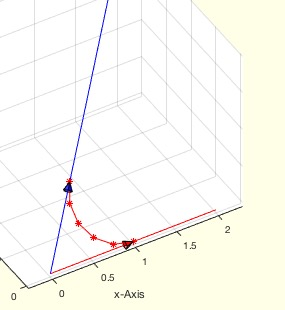% VLtangentcirc(v1,v2,R,M) - creates a vertex list for a tangential circle segment% (by Tim Lueth, VLFL-Lib, 2017-FEB-01 as class: ANALYTICAL GEOMETRY)% % This fnctn is used in VLradialedges (Status of: 2017-02-01)% % See also: VLradialEdges, PLtangentcirc% % VL=VLtangentcirc([v1,v2,R,M])% === INPUT PARAMETERS ===% v1: vector 1 from the origin% v2: vector 2 from the origin% R: desired Radius; automatically reduced; default is 1% M: maximize radius if possible; default is false% === OUTPUT RESULTS ======% VL: Vertex list describing a circle segment% % EXAMPLE: Try different parameters% VLtangentcirc% VLtangentcirc([5 0 0],[5 5 5],0.5)% VLtangentcirc([5 0 0],[5 5 5],0.5,true)%
###### FLwallofCPL(CPL,z)- returns only the wall and the cover wrt a CPL% FLwallofCPL(CPL,z) - returns only the wall and the cover wrt a CPL% (by Tim Lueth, VLFL-Lib, 2017-FEB-01 as class: SURFACES)% % In contrast to VLFLof CPLz, this fnctn returns only the faces of the % wall between two nested CPLs% % WORK IN PROGRESS (Status of: 2017-02-03)% % [FLW,FLT,CPL,VL]=FLwallofCPL(CPL,[z])% === INPUT PARAMETERS ===% CPL: Closed Polygon List% z: optional z value% === OUTPUT RESULTS ======% FLW: Facets of the Wall% FLT: Facets of the Top% CPL: Corrected Closed Polygon Line (nested but open)% VL: Vertex list%
###### PLtangentcirc(p1,p2,p3,R,M)- returns the a circ segment between p1 and p3 with radius R% PLtangentcirc(p1,p2,p3,R,M) - returns the a circ segment between p1 and p3 with radius R% (by Tim Lueth, VLFL-Lib, 2017-JAN-31 as class: ANALYTICAL GEOMETRY)% % See also: VLradialEdges, VLtangentcirc% % [PLS,pc]=PLtangentcirc([p1,p2,p3,R,M])% === INPUT PARAMETERS ===% p1: 1st point; alternatively PL% p2: 2nd point; alternatively desired radius% p3: 3rd point;; alternatively maximize radius; % R: Desired Radius% M: maximize radius; default is false% === OUTPUT RESULTS ======% PLS: Point List to replace 2nd point of the list% pc: % % EXAMPLE: try different examples% PLtangentcirc (CPLsample(1),5);%
###### VLsample(Nr,T)- returns a sample vertex list for testing algorithms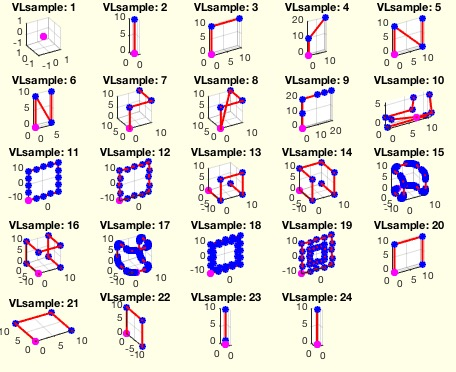% VLsample(Nr,T) - returns a sample vertex list for testing algorithms% (by Tim Lueth, VLFL-Lib, 2017-JAN-31 as class: MODELING PROCEDURES)% % See also: CPLsample, SGsample, PLsample% % [VL,PL]=VLsample([Nr,T])% === INPUT PARAMETERS ===% Nr: Nr of sample solid; default is none% T: Parameter for VLtrans (Value, Vector, Matrix)% === OUTPUT RESULTS ======% VL: Solid geometry% PL: Point list%